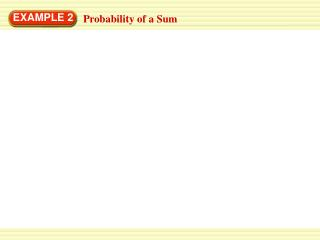DownloadDownload PresentationEXAMPLE 2

EXAMPLE 2

Download PresentationEXAMPLE 2

- - - - - - - - - - - - - - - - - - - - - - - - - - - E N D - - - - - - - - - - - - - - - - - - - - - - - - - - -
Presentation Transcript

1. EXAMPLE 2 Probability of a Sum

2. EXAMPLE 2 Probability of a Sum The spinners at the right are each divided into equal parts. You spin the spinners. Find the probability that the sum is at least 4.

3. EXAMPLE 2 Probability of a Sum The spinners at the right are each divided into equal parts. You spin the spinners. Find the probability that the sum is at least 4. SOLUTION You can use a table of sums to list All the possible outcomes

4. EXAMPLE 2 Probability of a Sum The spinners at the right are each divided into equal parts. You spin the spinners. Find the probability that the sum is at least 4. SOLUTION You can use a table of sums to list All the possible outcomes

5. EXAMPLE 2 Probability of a Sum The spinners at the right are each divided into equal parts. You spin the spinners. Find the probability that the sum is at least 4. SOLUTION You can use a table of sums to list All the possible outcomes 9 of the 12 sums are at least 4.

6. EXAMPLE 2 Probability of a Sum The spinners at the right are each divided into equal parts. You spin the spinners. Find the probability that the sum is at least 4. SOLUTION You can use a table of sums to list All the possible outcomes 9 of the 12 sums are at least 4. ANSWER The probability that the sum is at least 4 is . 3 9 = 4 12

7. EXAMPLE 3 Three Independent Events

8. EXAMPLE 3 Three Independent Events Games You are playing a game in which 3 canes are tossed. One side of each cane is flat, and the other side is round. Find the probability that all 3 canes land the same side up.

9. EXAMPLE 3 Three Independent Events Games You are playing a game in which 3 canes are tossed. One side of each cane is flat, and the other side is round. Find the probability that all 3 canes land the same side up. SOLUTION You can use a tree diagram to find all the possible outcomes.

10. EXAMPLE 3 Three Independent Events

11. EXAMPLE 3 Three Independent Events The probability that all 3 canes land the same side up is . ANSWER 2 1 = 8 4

12. for Examples 2 and 3 GUIDED PRACTICE Find the probability of the event. 4. The sum in Example 2 is at most 4. ANSWER 5 of the 12 sums are at most 4. The probability that the sum is at most 4 is . 5 12

13. R R R R R F R F R R F F F R R F R F F F R F F F for Examples 2 and 3 GUIDED PRACTICE 5. Exactly one cane in Example 3 lands flat side up. ANSWER 3 . Out of 8, 3 canes lands flat side up once. So solution is 8